# MAFS.912.G-CO.2.8Archived StandardExport Print
Explain how the criteria for triangle congruence (ASA, SAS, SSS, and Hypotenuse-Leg) follow from the definition of congruence in terms of rigid motions.
General Information
Subject Area: Mathematics
Domain-Subdomain: Geometry: Congruence
Cluster: Level 2: Basic Application of Skills & Concepts
Cluster: Understand congruence in terms of rigid motions. (Geometry - Major Cluster) -

Clusters should not be sorted from Major to Supporting and then taught in that order. To do so would strip the coherence of the mathematical ideas and miss the opportunity to enhance the major work of the grade with the supporting clusters.

Date of Last Rating: 02/14
Status: State Board Approved - Archived
Assessed: Yes
Test Item Specifications
Assessed with:
MAFS.912.G-CO.2.6

Sample Test Items (1)
• Test Item #: Sample Item 1
• Question:

Triangles BKW and DMT are shown where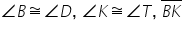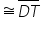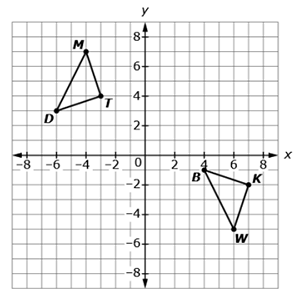This question has three parts.

Rashhaad performs a sequence of transformations on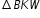to map it to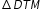.

Part A. Which sequence of transformations could be used to mapto?

Part B. The coordinate grid below shows three triangles that could be an intermediate step of Rashaad's sequence of transformations.

Step 1: Use the Add Arrow tool to map the vertices ofto show the translation (s) chosen in part A.

Step 2: Then, label the transformed triangle by dragging B',K', and W' to the correct vertex.

Part C.

Rashaad wants to justify thatare sufficient to show that the triangles are congruent.

Select words, phrases or equations to complete Rashaad's justification.

• Difficulty: N/A
• Type: : Multiple Types

## Related Courses

This benchmark is part of these courses.
1200400: Foundational Skills in Mathematics 9-12 (Specifically in versions: 2014 - 2015, 2015 - 2022, 2022 and beyond (current))
1206300: Informal Geometry (Specifically in versions: 2014 - 2015, 2015 - 2022 (course terminated))
1206310: Geometry (Specifically in versions: 2014 - 2015, 2015 - 2022, 2022 and beyond (current))
1206320: Geometry Honors (Specifically in versions: 2014 - 2015, 2015 - 2022, 2022 and beyond (current))
7912060: Access Informal Geometry (Specifically in versions: 2014 - 2015 (course terminated))
1206315: Geometry for Credit Recovery (Specifically in versions: 2014 - 2015, 2015 - 2022, 2022 and beyond (current))
7912065: Access Geometry (Specifically in versions: 2015 - 2022, 2022 and beyond (current))

## Related Access Points

Alternate version of this benchmark for students with significant cognitive disabilities.

## Related Resources

Vetted resources educators can use to teach the concepts and skills in this benchmark.

## Formative Assessments

Justifying HL Congruence:

Students are asked to use rigid motion to explain why the HL pattern of congruence ensures right triangle congruence.

Type: Formative Assessment

Justifying SSS Congruence:

Students are asked to use rigid motion to explain why the SSS pattern of congruence ensures triangle congruence.

Type: Formative Assessment

Justifying SAS Congruence:

Students are asked to use rigid motion to explain why the SAS pattern of congruence ensures triangle congruence.

Type: Formative Assessment

Justifying ASA Congruence:

Students are asked to use rigid motion to explain why the ASA pattern of congruence ensures triangle congruence.

Type: Formative Assessment

## Lesson Plans

Turning to Congruence:

This lesson will use rigid motions to prove the ASA and HL triangle congruence theorems.

Type: Lesson Plan

Analyzing Congruence Proofs:

This lesson is intended to help you assess how well students are able to:

• Work with concepts of congruency and similarity, including identifying corresponding sides and corresponding angles within and between triangles.
• Identify and understand the significance of a counter-example.
• Prove, and evaluate proofs in a geometric context.

Type: Lesson Plan

Why Does ASA Work?:

This problem solving task ask students to show the reflection of one triangle maps to another triangle.

When Does SSA Work to Determine Triangle Congruence?:

In this problem, we considered SSA. The triangle congruence criteria, SSS, SAS, ASA, all require three pieces of information. It is interesting, however, that not all three pieces of information about sides and angles are sufficient to determine a triangle up to congruence.

Why Does SAS Work?:

This problem solving task challenges students to explain the reason why the given triangles are congruent, and to construct reflections of the points.

Why does SSS work?:

This particular problem solving task exhibits congruency between two triangles, demonstrating translation, reflection and rotation.

Are the Triangles Congruent?:

The purpose of this task is primarily assessment-oriented, asking students to demonstrate knowledge of how to determine the congruency of triangles.

## Tutorials

Finding congruent triangles:

In this tutorial, students will use the SSS, ASA, SAS, and AAS postulates to find congruent triangles

Type: Tutorial

Using SSS in a proof:

This tutorial discusses the difference between a theorem and axiom. It also shows how to use SSS in a proof.

Type: Tutorial

Triangle congruence postulates:

This tutorial discusses SSS, SAS, ASA and AAS postulates for congruent triangles. It also shows AAA is only good for similarity and SSA is good for neither.

Type: Tutorial

Congruent Triangles and SSS:

In this video, students will learn about congruent triangles and the "Side-Side-Side" postulate.

Type: Tutorial

## Worksheet

Students are provided two pairs of quadrilaterals with information that relates those in each of the pairs. The objective is to prove that each of the pairs are congruent. The teacher will provide guidance to the students as necessary by suggesting a triangle congruence theorem and/or construction of a diagonal.

Type: Worksheet

## MFAS Formative Assessments

Justifying ASA Congruence:

Students are asked to use rigid motion to explain why the ASA pattern of congruence ensures triangle congruence.

Justifying HL Congruence:

Students are asked to use rigid motion to explain why the HL pattern of congruence ensures right triangle congruence.

Justifying SAS Congruence:

Students are asked to use rigid motion to explain why the SAS pattern of congruence ensures triangle congruence.

Justifying SSS Congruence:

Students are asked to use rigid motion to explain why the SSS pattern of congruence ensures triangle congruence.

## Student Resources

Vetted resources students can use to learn the concepts and skills in this benchmark.

Why Does ASA Work?:

This problem solving task ask students to show the reflection of one triangle maps to another triangle.

When Does SSA Work to Determine Triangle Congruence?:

In this problem, we considered SSA. The triangle congruence criteria, SSS, SAS, ASA, all require three pieces of information. It is interesting, however, that not all three pieces of information about sides and angles are sufficient to determine a triangle up to congruence.

Why Does SAS Work?:

This problem solving task challenges students to explain the reason why the given triangles are congruent, and to construct reflections of the points.

Why does SSS work?:

This particular problem solving task exhibits congruency between two triangles, demonstrating translation, reflection and rotation.

Are the Triangles Congruent?:

The purpose of this task is primarily assessment-oriented, asking students to demonstrate knowledge of how to determine the congruency of triangles.

## Tutorials

Finding congruent triangles:

In this tutorial, students will use the SSS, ASA, SAS, and AAS postulates to find congruent triangles

Type: Tutorial

Using SSS in a proof:

This tutorial discusses the difference between a theorem and axiom. It also shows how to use SSS in a proof.

Type: Tutorial

Triangle congruence postulates:

This tutorial discusses SSS, SAS, ASA and AAS postulates for congruent triangles. It also shows AAA is only good for similarity and SSA is good for neither.

Type: Tutorial

Congruent Triangles and SSS:

In this video, students will learn about congruent triangles and the "Side-Side-Side" postulate.

Type: Tutorial

## Parent Resources

Vetted resources caregivers can use to help students learn the concepts and skills in this benchmark.

Why Does ASA Work?:

This problem solving task ask students to show the reflection of one triangle maps to another triangle.

When Does SSA Work to Determine Triangle Congruence?:

In this problem, we considered SSA. The triangle congruence criteria, SSS, SAS, ASA, all require three pieces of information. It is interesting, however, that not all three pieces of information about sides and angles are sufficient to determine a triangle up to congruence.

Why Does SAS Work?:

This problem solving task challenges students to explain the reason why the given triangles are congruent, and to construct reflections of the points.